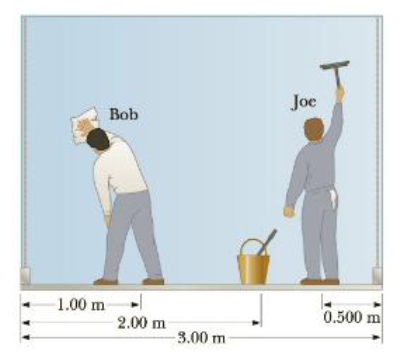Chapter 8, Problem 82AP

Chapter
Section
Textbook Problem

Two window washers. Bob and Joe, are on a 3.00-m-long, 345-N scaffold supported by two cables attached to its ends. Bob weighs 750 N and stands 1.00 m from the left end, as shown in Figure P8.82. Two meters from the left end is the 500-N washing equipment. Joe is 0.500 m from the right end and weighs 1 000 N. Given that the scaffold is in rotational and translational equilibrium, what are the forces on each cable?Figure P8.82

To determine
The forces on each cable.

Explanation

Given Info:

Weight of the bob is 750 N and he is 1.00 m from the left end, weight of the Joe is 1000 N and he is 0.500 m from the right end, weight of the washing equipment 500 N and it is 2.00 m from the left end, weight of the scaffold 345 and it acts at the midpoint 1.5 m of the scaffold of length 3.00 m.

The scaffold itself is in rotational as well as translational equilibrium therefore total external torque on the scaffold is zero. Consider outward torque as positive and inward torque as negative.

Consider an axis passes into the page of the left side scaffold

Figure shows the forces acting on the scaffold, weight of the Bob and Joe, weight of the washing equipment, and forces on each cables at left end and right end

• WB is the weight of the Bob
• WS is the weight of the scaffold
• WJ is the weight of the Joe
• WE is the weight washing equipment
• TR is the force on the right cable

The equilibrium condition on the cable is,

τ=0

Use the condition of equilibrium to write the torque equation for the situation shown in the above figure.

WB(1.00m)WS(1.5m)WE(2.00m)WJ(2.50m)+TR(3.00m)=0

Substitute, 750N for WB , 500N for WE , 345N for WS , 1000N for WJ , to calculate TR .

{(750N)(1.00m)(500N)(2.00m)(345N)(1.50m)(1000N)(2

Still sussing out bartleby?

Check out a sample textbook solution.

See a sample solution

The Solution to Your Study Problems

Bartleby provides explanations to thousands of textbook problems written by our experts, many with advanced degrees!

Get Started